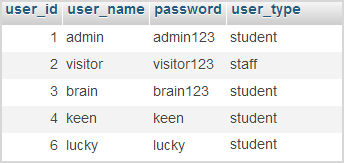# MySQL Fetch using PHP

PHP provides a huge list of MySQL functions to access the database from the front end. Among those functions, we are going to discuss some of them that are used to fetch data from the database. Such functions differ with the type of results they are expected to return.

In PHP, MySQL fetches results can be obtained by the following functions. All of these functions will fetch only one row per function call. If required, we should call such functions with a loop for getting subsequent MySQL fetch results row by row.

• mysqli_fetch_row()
• mysqli_fetch_assoc()
• mysqli_fetch_array()
• mysqli_fetch_object()
• mysqli_fetch_lengths()
• mysqli_fetch_field()

## mysqli_fetch_row()

This function will fetch data about the single row with which the row pointer currently exists. After fetching the entire row details, it will be returned as an array with number indices corresponding to the MySQL field offset.

The mysqli_fetch_row() function requires a resource data that is returned by executing query appropriate with MySQL fetch operations. If no results found for the query, then mysqli_fetch_row() will return NULL.

Let us consider the Users table, which we had taken for example while seeing about MySQL Left JOIN and MySQL Right JOIN. And the table data and structure is as follows.The following PHP program is for fetching MySQL data using mysqli_fetch_row(). Before that, the database connections are made with first two lines as usual.

```\$conn = mysqli_connect("localhost", "root", "test", "blog_samples") or die("Connection Error: " . mysqli_error(\$conn));
\$query = "SELECT * from Users";
\$result = mysqli_query(\$conn, \$query) or die(mysqli_error(\$conn));
\$row = mysqli_fetch_row(\$result);
print "<pre>";
print_r(\$row);
print "<pre>";
```

This program will return values of the entire first row since the row pointer is at the beginning. So the output will be as shown below that can be cross checked with the table data shown already.

```Array
(
 => 1
 => student
)
```

If we want to get all the row information, we should do the same process recursively by iterating through a loop until end of the record. For example,

```while(\$row = mysqli_fetch_row(\$result)) {
print "<pre>";
print_r(\$row);
print "<pre>";
}
```

## mysqli_fetch_assoc()

This function is similar to the mysqli_fetch_row(), except that, it will return an array of row information containing column values are indexed with the column name. So the result type is an associative array where each column name and values of a single row are associated together as name, value pairs.

Let us replace mysqli_fetch_row() with mysqli_fetch_assoc() in the above program which will return the following array.

```Array
(
[user_id] => 1
[user_type] => student
)
```

By using this type of MySQL fetch, we can access the data by its name instead of its offset. Since, remembering the order of fields is too tough for a huge list of columns, accessing by field name is easy and there by this function could be preferred in such scenario.

## mysqli_fetch_array()

This MySQL fetch method returns resultant array with both indices, that is, field offset and field name. So, it would be used most probably by having both option of indexing.Unlike above two functions, mysqli_fetch_array() accepts an optional argument for specifying resultant array index type and its possible values are,

• MYSQLI_BOTH – It is the default value that would be taken if no second argument is provided for this function. It will provide resultant array with both indices.
• MYSQLI_NUM – With this option, mysqli_fetch_array() will return array with offset indices as same as mysqli_fetch_row().
• MYSQLI_ASSOC – With this option, mysqli_fetch_array() will return array with name indices as same as mysqli_fetch_assoc().

By replacing mysqli_fetch_row() with mysqli_fetch_array(), the output array will be,

```Array
(
 => 1
[user_id] => 1
 => student
[user_type] => student
)
```

## mysqli_fetch_object()

mysqli_fetch_object() function will return MySQL data with same structure as returned by mysqli_fetch_assoc(), but its type is different. mysqli_fetch_object() returns object where as mysqli_fetch_assoc() returns array. So, the way of accessing these data will also be differed. For example, if we are required to access user_name, after array fetch, it will be done by,

```echo \$row["user_name"];
```

Or else after object fetch, it would be,

```echo \$row->user_name;
```

## mysqli_fetch_lengths()

This PHP function is used to return the string length each column value of the recently fetched row. So, before calculating the string length, any one of the above MySQL fetch functions need to be invoked.

For example, the following program is to fetch single row data using mysqli_fetch_object() and to print the corresponding length array to the browser.

```\$conn = mysqli_connect("localhost", "root", "test", "blog_samples") or die("Connection Error: " . mysqli_error(\$conn));

\$query = "SELECT * from Users";
\$result = mysqli_query(\$conn, \$query) or die(mysqli_error(\$conn));
\$row = mysqli_fetch_object(\$result);
\$student_length = mysqli_fetch_lengths(\$result);
print "<pre>";
print_r(\$row);
print_r(\$student_length);
print "<pre>";
```

And the output is,

```stdClass Object
(
[user_id] => 1
[user_type] => student
)
Array
(
 => 1
 => 5
 => 8
 => 7
)
```

Obviously, the object properties array and the length array are returned as shown above that could be cross checked manually to ensure the property value length is correct as expected.

## mysqli_fetch_field()

Unlike above functions, mysqli_fetch_field() is for getting MySQL Database table’s field information instead of record data. And, this function also fetch one field per call and need loop implementation for getting more fields. These information array will be returned as an object which includes properties like, table name, field name, field maximum length, primary key flag offset and etc. For example, the user_id field details of Users table is returned as follows.

```stdClass Object
(
[name] => user_id
[table] => Users
[def] =>
[max_length] => 1
[not_null] => 1
[primary_key] => 1
[multiple_key] => 0
[unique_key] => 0
[numeric] => 1
[blob] => 0
[type] => int
[unsigned] => 0
[zerofill] => 0
)
```

## Comments to “MySQL Fetch using PHP”

• Rajeshri Anant Pevekar says:

nice